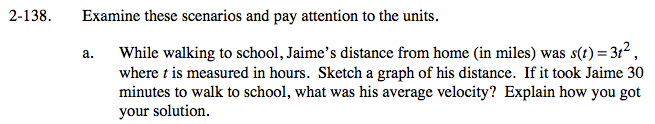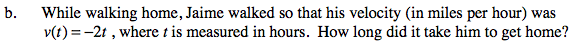### Home > CALC > Chapter 2 > Lesson 2.4.1 > Problem2-138

2-138.This is a distance graph. Time, t, should be on the x-axis. (It usually is!) And distance, s(t), should be on the y-axis. Sketch the graph of s(t) = 3t².

Note that this is the slope of the secant line that connects the points at t = 0 and t = 5.Sketch a velocity graph of v(t) = −2t. Time, t, will be on the x-axis. Velocity, v(t), will be on the y-axis. How can distance information be found on a velocity graph?